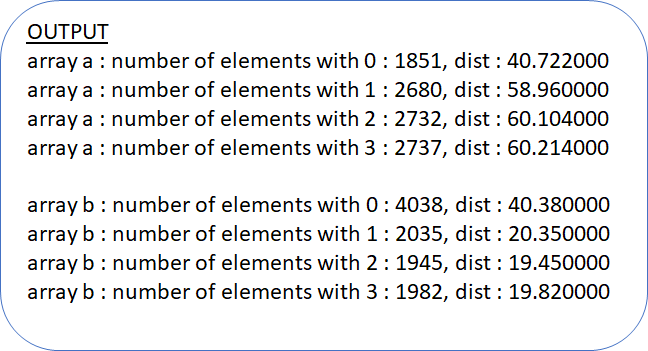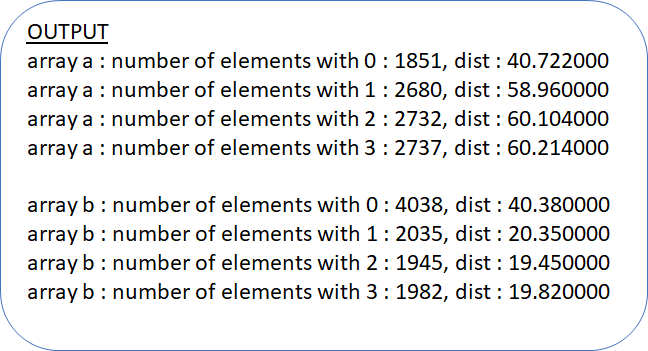# Trung tâm đào tạo thiết kế vi mạch Semicon

• Đăng ký
 Name: * Tên đăng nhập: * Email: * Mật khẩu: * Verify Password: * Fields marked with an asterisk (*) are required.## WEIGHTED DISTRIBUTION IN SYSTEM VERILOGIn constraint random verification, it may take a long time for a particular corner case to be generated which scenario we never thought. Sometimes even after running test-case regression for N number of time corner case may not be generated and you may see holes in functional coverage.

To resolve this issue you can use a weighted distribution to drive stimulus in particular manner.

The dist keyword in SystemVerilog allows you to create weighted distributions so that some values are chosen more often than others. There are 2 different kinds of distribution operators available in SystemVerilog.

The:= operator assigns the specified weight to the item or, if the item is a range, to every value in the range.

The :/ the operator assigns the specified weight to the item or, if the item is a range, to the range as a whole. If there are n values in the range, the weight of each value is range_weight / n.

Let’s go through the below example to understand how weighted distribution works

class weight_dist_class;

rand bit [1:0] a;

rand bit [1:0] b;

constraint dist_a_cn {

a dist {0     := 40,

[1:3] := 60};

// a = 0, weight = 40/(40+60+60+60) = 40/220

// a = 1, weight = 60/220

// a = 2, weight = 60/220

// a = 3, weight = 60/220

}

constraint dist_b_cn {

b dist {0     :/ 40,

[1:3] :/ 60};

// b = 0, weight = 40/(40+60) = 40/100

// b = 1, weight = (60/3)/100 = 20/100

// b = 2, weight = (60/3)/100 = 20/100

// b = 3, weight = (60/3)/100 = 20/100

}

endclass : weight_dist_class

program main;

weight_dist_class dist_c;

bit [1:0] a;

bit [1:0] b;

bit [1:0] temp_q[\$];

initial begin

dist_c = new();

for (int i=0; i<10000 ; i++) begin

if (!dist_c.randomize()) begin

\$error("Randomization failed");

end

a[i] = dist_c.a;

b[i] = dist_c.b;

end

for(int i=0; i<4; i++) begin

temp_q = a.find with (item == i);

\$display("array a : number of elements with %0d : %0d, dist : %f",

i, temp_q.size(), (real'(temp_q.size())*220)/10000 );

end

\$display();

for(int i=0; i<4; i++) begin

temp_q = b.find with (item == i);

\$display("array b : number of elements with %0d : %0d, dist : %f",

i, temp_q.size(), (real'(temp_q.size())*100)/10000 );

end

end

endprogram : mainBạn Có Đam Mê Với Vi Mạch hay Nhúng      -     Bạn Muốn Trau Dồi Thêm Kĩ Năng

Mong Muốn Có Thêm Cơ Hội Trong Công Việc

Và Trở Thành Một Người Có Giá Trị Hơn

# Hãy Để Chúng Tôi Hỗ Trợ Cho Bạn. SEMICONLần cập nhật cuối ( Chủ nhật, 19 Tháng 9 2021 16:05 )